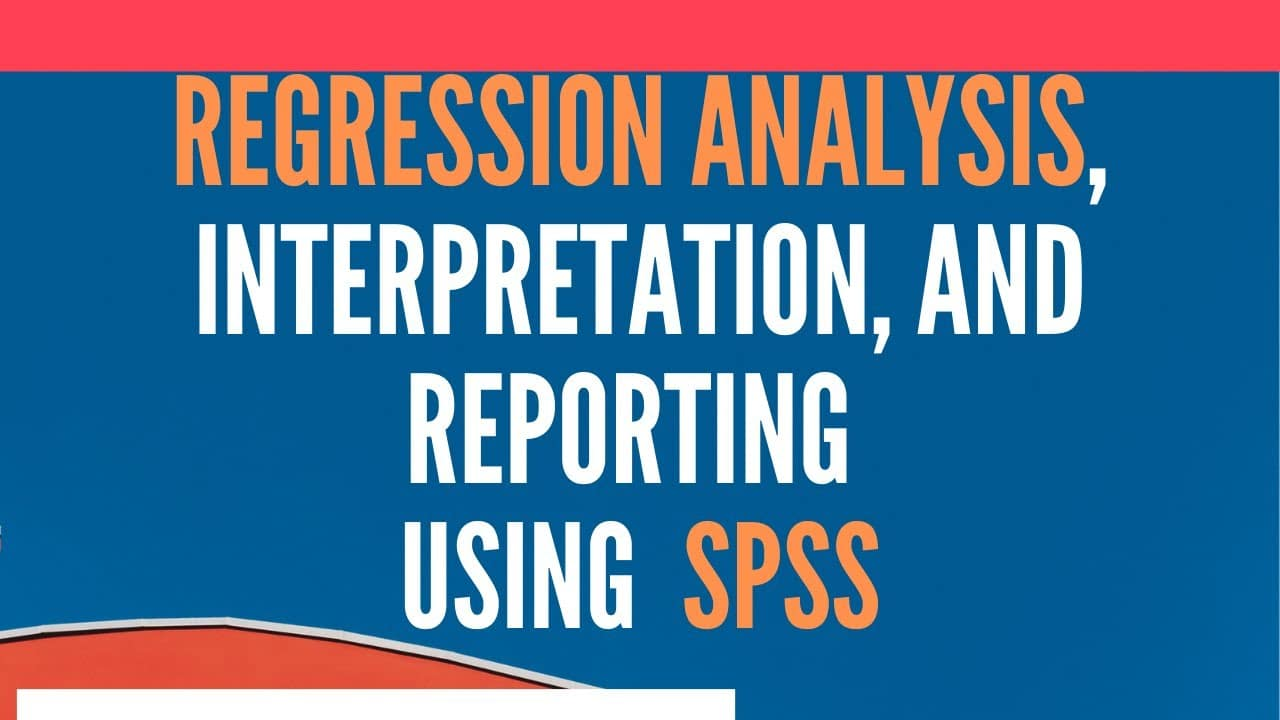# Regression Analysis using SPSS#### Regression Analysis using SPSS

The tutorial guides the researchers on the concept, interpretation, and reporting Linear and Multiple Regression using SPSS.

Tutorials include

1. Concept of Regression Analysis.
2. Differences between Correlation and Regression Analysis.
3. Regression Examples
4. Bivariate and Multiple Regression
5. Interpreting and Reporting Regression Results

## The Concept of Regression Analysis using SPSS

Regression technique is used to assess the strength of a relationship between one dependent and independent variable(s). It helps in predicting value of a dependent variable from one or more independent variable. Regression analysis helps in predicting how much variance is being accounted in a single response (dependent variable) by a set of independent variables.

This session looks into two kinds of regression

• Bivariate Regression
• Multiple Regression

Bivariate regression is similar to bivariate correlation, because both are designed for situations in which there are just two variables. Multiple regression, however, was created for cases in which there are three or more variables. The different kinds of regression are like correlation, since they are utilized to evaluate the relationship among variables. This may make you think that regression is another way to measure correlation, however there are difference in correlation and regression in their purpose, labeling of variables and the kinds of inferential tests applied.

### Differences between Correlation and Regression Analysis

 Characteristics Correlation Regression Purpose of Technique Association/Connection between two variables Understanding Link i-e Prediction and Explanation Labels Attached No Clear labels Clear Distinction of Dependent and Independent Variable Inferential Tests Correlation Coefficient Regression Coefficient, Intercept, t-statistics, Change in Regression Coefficient.

### Regression Examples

Following are few scenarios in which we could use Regression Analysis

• The sales manager would like to assess if sales force training results in reduction in losses.
• The HR manager would like to know if increase in salary leads to low turnover.
• The management would like to know if a particular leadership style helps in improving career satisfaction.
• A manager in non-governmental organization would like to know if community hostility has an impact on project success.
• How much of the variance in life satisfaction scores can be explained by the following set of variables: servant leadership, career satisfaction, job satisfaction, internal marketing, and role stress.?

## Bivariate Regression Analysis using SPSS

The simplest of regression analysis is called bivariate regression. Two variables are involved in the bivariate regression. One is the Dependent variable that is to be predicted, the other is independent variable that explains the variance in the dependent variable. The purpose of this kind of regression can be either prediction or explanation; however, bivariate regression is most frequently used to see how well scores on the dependent variable can be predicted from data on the independent variable.

Predicting the value of a variable from one or more other variables

Regression analysis is used to predict the values of a dependent variable given the values of one or more independent variables by calculating a regression equation. For instance a marketing manager would like to predict If variation in sales can be explained in terms of variation in advertising expenditure?

Yi = β0 +β1Xi +e

In case of the above scenario the equation can be modified as follows

Sales = β0 + β1(Advertising Budget) + e

Where

Sales is the dependent variable that is to be predicted

β0 is the regression constant, Representing a value of sales if the company indulges in no advertising.

β1 is the beta coefficient

e represents error terms that simply speaking accounts for all other factors that account variance in the dependent variable.

## Important Terms in Regression

Regression Coefficient

• Regression coefficient is a measure of how strongly each IV (also known as predictor variable) predicts the DV.

R Values

• This is the correlation coefficient. Regression analysis would provide you with two different R values. A simple R value represents the correlation between the observed values and the predicted values (based on the regression equation obtained) of the DV. The other R values is referred to as R Square. R square shows how much variance in the dependent variable is being explained by the indepenent variable(s). For example, R Square value of 0.70 would mean that the IVs in the model can predict 70% of the variance in the DV.

## The Concept of Multiple Regression

• From a single independent variable, now we move our attention to more than one independent variable. This form of regression involves, like bivariate regression, a single dependent variable.
• In multiple regression, however, there are two or more independent variables. Stated differently, multiple regression involves just one Y variable but two, three, or more X variables. Multiple regression is utilized when there is more than 1 independent variable.

## Reporting Regression Analysis using SPSS

The Problem:

To investigate if Servant Leadership has a significant impact on life satisfaction.

Hypothesis

H1: There is a significant impact of servant leadership on life satisfaction.

The hypothesis tests if servant leadership carries a significant impact on life satisfaction. The dependent variable LS was regressed on predicting variable SL to test hypothesis H1. SL significantly predicted LS, F (1, 219) = 83.599, p < 0.001, which indicates that the SL can play a significant role in shaping LS (b = .579, p < .001). These results clearly direct the positive effect of the SL. Moreover, the R2 = .276 depicts that the model explains 27.6% of the variance in LS. The table shows the summary of the findings.

 Hypothesis Regression Weights Beta Coefficient R2 F t-value p-value Hypotheses Supported H1 SL → LS .579 .276 83.599 .000 Yes

Note: *p < 0.05. SL: Servant Leadership, LS: Life Satisfaction